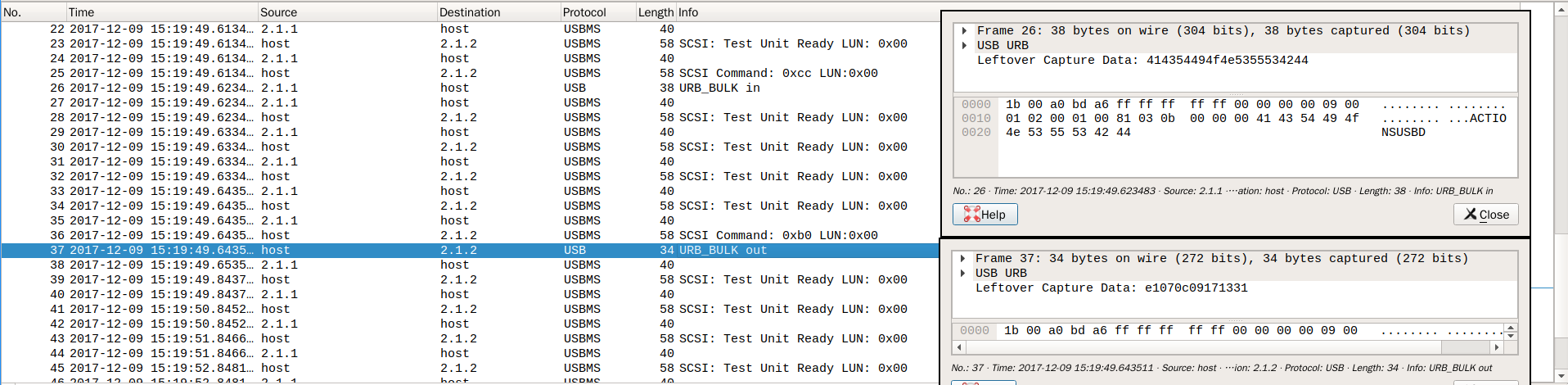12
10
2017

### 在 Linux 下设置录音笔的时间

Wine 是不行的，硬件相关的东西基本上没戏。拿 Procmon 跟踪了一下，也没什么复杂的操作，主要部分就几个 DeviceIoControl 调用，但是看不到调用参数。试了试 IDA，基本看不懂……不过倒是能知道，它通过 IOCTL_SCSI_PASSTHROUGH 直接给设备发送了 SCSI 命令。```#!/usr/bin/env python3

import os
import sys
import struct
import subprocess
import datetime

def set_time(dev):
cmd = ['sg_raw', '-s', '7', dev, 'b0', '00', '00', '00', '00', '00',
'00', '07', '00', '00', '00', '00']
p = subprocess.Popen(cmd, stdin=subprocess.PIPE, stderr=subprocess.PIPE)
dt = datetime.datetime.now()
data = struct.pack('<HBBBBB', dt.year, dt.month, dt.day,
dt.hour, dt.minute, dt.second)
_, stderr = p.communicate(data)
ret = p.wait()
if ret != 0:
raise subprocess.CalledProcessError(ret, cmd, stderr=stderr)

def actionsusbd(dev):
cmd = ['sg_raw', '-r', '11', dev, 'cc', '00', '00', '00', '00', '00',
'00', '0b', '00', '00', '00', '00']
subprocess.run(cmd, check=True, stderr=subprocess.PIPE)

def main():
if len(sys.argv) != 2:
sys.exit('usage: setudisktime DEV')

dev = sys.argv
if not os.access(dev, os.R_OK | os.W_OK):
sys.exit(f'insufficient permission for {dev}')

actionsusbd(dev)
set_time(dev)

if __name__ == '__main__':
main()
```
Category: Linux | Tags: linux 硬件 usb scsi

| Theme: Aeros 2.0 by TheBuckmaker.com